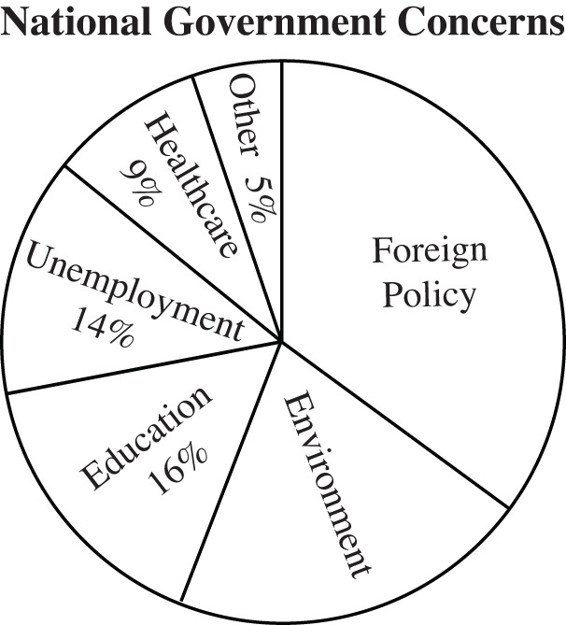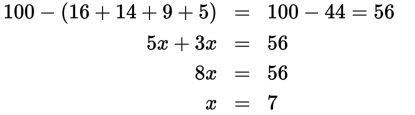# SAT Math Multiple Choice Question 485: Answer and Explanation

### Test Information

Question: 485

5.Margo surveyed all the students in the government classes at her school to see what they thought should be the most important concern of a national government. The results of the survey are shown in the figure above. If the ratio of students who answered "Foreign Policy" to those who answered "Environment" was 5:3, what percentage of the students answered "Environment"?

• A. 16%
• B. 21%
• C. 24%
• D. 35%

Explanation:

B

Difficulty: Easy

Category: Problem Solving and Data Analysis / Rates, Ratios, Proportions, and Percentages

Strategic Advice: Break the question into steps. Before you can use the ratio, you need to find the percent of the students who answered either "Foreign Policy" or "Environment."

Getting to the Answer: The ratio given in the question is 5:3, so write this as 5 parts "Foreign Policy"and 3 parts "Environment." You don't know how big a part is, so call it x. This means that 5x + 3x = the percent of the students who answered either "Foreign Policy" or "Environment," which is 100% – all the other answers:Each part has a value of 7, and 3 parts answered "Environment," so the correct percentage is 3(7) = 21%.